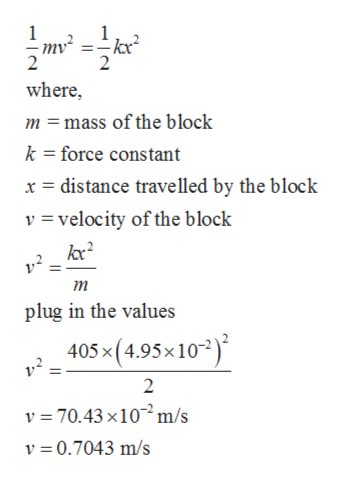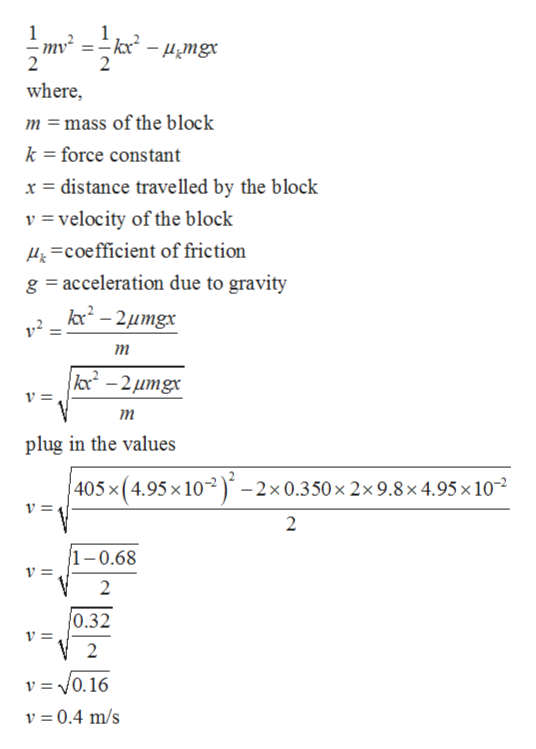# kwww.wmxxx0

Question

A block of mass m = 2.00 kg is attached to a spring of force constant k = 405 N/m as shown in the figure below. The block is pulled to a position xi = 4.95 cm to the right of equilibrium and released from rest.

(a) Find the speed the block has as it passes through equilibrium if the horizontal surface is frictionless.

(b) Find the speed the block has as it passes through equilibrium (for the first time) if the coefficient of friction between block and surface is μk = 0.350.

check_circle

Step 1

Given:

Mass of the block = 2.00 kg

Force constant = 405 N/m

Distance travelled by the block = 4.95 cm

Coefficient of friction, μk = 0.350

Step 2

For frictionless surface,

Speed of the block as it passes through equilibrium can be calculated as;

K.E. of the block = P.E. energy of the blockhelp_outlineImage Transcriptionclosewhere m mass of the block k force constant distance travelled by the block x v = velocity of the block т plug in the values 405x(4.95x10) 2 v 70.43x10m/s v 0.7043 m/s fullscreen
Step 3

Speed of the block as it passes through equilibrium and μk is the coeff...help_outlineImage Transcriptionclosewhere, m =mass of the block k =force constant x distance travelled by the block v=velocity of the block &Coefficient of friction g acceleration due to gravity kc2-2umgx тm kc-2umgx т plug in the values 405x (4.95 x10-2x 0.350 x 2x 9.8 x 4.95 x 10 v = 2 1-0.68 2 0.32 v =0.16 v 0.4 m/s fullscreen

### Want to see the full answer?

See Solution

#### Want to see this answer and more?

Solutions are written by subject experts who are available 24/7. Questions are typically answered within 1 hour.*

See Solution
*Response times may vary by subject and question.
Tagged in

### Friction